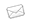# COURSE: BSC IN NATURAL SCIENCE (PHYSICS)N/A
###### Duration:
3 Years Full Time / Full Time / Part Time
After completing this programme, students will be familiar with all the main branches of physics, including quantum, solid state and atomic and nuclear physics. They will also have a good understanding of related mathematics disciplines such as linear algebra and calculus. Practical assignments and projects are an integral part of the programme, ensuring that students’ knowledge goes beyond the purely theoretical.

Duration: 3 years part time

#### Compulsory Subjects

##### First year subjects:

• Modern physics

• Practical work

• Linear algebra

• Pre-calculus Mathematics

• Calculus

• General chemistry

• Comprehension skills for science

• End-user Computing

##### Second year subjects:

• Classical mechanics

• Electricity and magnetism

• Waves

• Calculus in higher dimensions

• Linear algebra

• Differential equations

##### Third year subjects:

• Quantum physics

• Solid state physics

• Atomic and nuclear physics

• Mechanics and the calculus of variations

• Statistical physics

• Complex analysis

• Ordinary differential equations

Careers: physical modeller, mathematical modeller, teacher, entrepreneur.

Source: www.unisa.ac.za

AVAILABLE BURSARIES
No related bursaries found
RELATED COURSES
No related courses found